# NCERT Solutions Class 9 Maths Chapter 2 Polynomial

Ncert Solutions for class 9 subject Maths Chapter 2 Polynomialin pdf Best Free NCERT Solutions for class 1 to 12 in pdf NCERT Solutions, cbse board, Maths, ncert Solutions for Class 9 Maths, class 9 Maths ncert solutions, Polynomial, Class 9, ncert solutions chapter 2 Polynomial, class 9 Maths, class 9 Maths ncert solutions, Maths ncert solutions class 9, Ncert Solutions Class 9 Mathematics Chapter 2 Polynomial

## Concept Chapter 2 Class 9 Maths Polynomial Ncert Solutions

What is a Polynomial?

Polynomial p(x) in one variable x is an algebraic expression in x of the form
p(x) = anxn + an–1xn – 1 + . . . + a2x2 + a1x + a0,
where a0, a1, a2, . . ., an are constants and an ≠ 0.
a0, a1, a2, . . ., an are respectively the coefficients of x0, x, x2, . . ., xn,
and n is called the degree of the polynomial.
Each of anxn, an–1 xn–1, ..., a0, with an ≠ 0, is called a term of the polynomial p(x).

Type of Polynomial

A polynomial of one term is called a monomial.
Example p(x) = x

A polynomial of two terms is called a binomial.
Example q(x) = x2 – x,

A polynomial of three terms is called a trinomial.
Examples r(y) = y3 + y + 1

A polynomial of degree one is called a linear polynomial.
Example p(x) = x +1

A polynomial of degree two is called a quadratic polynomial.
Examples p(x) = x2 + x + 2

A polynomial of degree three is called a cubic polynomial.
Example r(y) = y3 + y + 1

Other Information
A real number ‘a’ is a zero of a polynomial p(x) if p(a) = 0. In this case, a is also called a root of the equation p(x) = 0.

Every linear polynomial in one variable has a unique zero, a non-zero constant polynomial has no zero, and every real number is a zero of the zero polynomial.

Remainder Theorem : If p(x) is any polynomial of degree greater than or equal to 1 and p(x) is ided by the linear polynomial x – a, then the remainder is p(a).

Factor Theorem : x – a is a factor of the polynomial p(x), if p(a) = 0. Also, if x – a is a factor of p(x), then p(a) = 0.

The highest power of the variable in a polynomial as the degree of the polynomial

The degree of a non-zero constant polynomial is zero.

Identity I : (x + y)2 = x2 + 2xy + y2

Identity II : (x – y)2 = x2 – 2xy + y2

Identity III : x2 – y2 = (x + y) (x – y)

Identity IV : (x + a) (x + b) = x2 + (a + b)x + ab
Identity V : (x + y + z)2 = x2 + y2 + z2 + 2xy + 2yz + 2zx
Identity VI : (x + y)3 = x3 + y3 + 3xy (x + y)
Identity VII : (x – y)3 = x3 – y3 – 3xy(x – y)
= x3 – 3x2y + 3xy2 – y3
Identity VIII : x3 + y3 + z3 – 3xyz = (x + y + z)(x2 + y2 + z2 – xy – yz – zx)

## Exercise 2.1 Chapter 2 Class 9 Maths Polynomial Ncert Solutions

Question 1. Which of the following expressions are polynomials in one variable and which are not? State reasons for your answer.
(i) 4x2 – 3x + 7 (ii) y2 + √2 (iii) 3√t + t√ 2 (iv) y +2/y (v) x10 + y3 + t50

Solution (i)4x2 – 3x + 7
there is only one variable x with whole number power so this polynomial in one variable

(ii)y2 + √2
there is only one variable y with whole number power so this polynomial in one variable

(iii) 3√t + t√ 2
there is only one variable t but in 3√t power of t is ½ which is not a whole number so 3√t + t√ 2 is not a polynomial

(iv)y +2/y
there is only one variable y but 2/y = 2y-1 so the power is not a whole number so y +2/y is not a polynomial

(v) x10 + y3 + t50
there are three variable x y and t and there powers are whole number so this polynomial in three variable

p>

Question 2. Write the coefficients of x2 in each of the following:
(i) 2 + x2 + x (ii) 2 – x2 + x3 (iii) (pi/2 )x2+ x (iv) √2x – 1
Solution: (i) 2 + x2 + x

Coefficient if x2 = 1

(ii) 2 – x2 + x3
Coefficient of x2 = -1

(iii) (pi/2 )x2+ x
Coefficient of x2 = pi/2

(iv) √2x – 1
There are no x2 term so Coefficient of x2 = 0

Question 3. Give one example each of a binomial of degree 35, and of a monomial of degree 100.

Solution example of a binomial of degree 35
degree is 35 so maximum power of term will 35 and it is binomial so it has 2 terms
so such example is x35 + 1
example of a monomial of degree 100
degree is 100 so maximum power of term will 100 and it is monomial so it has 1 terms
so such example is x100

p>

Question 4. Write the degree of each of the following polynomials:
(i) 5x3 + 4x2 + 7x (ii) 4 – y2 (iii) 5t – √7 (iv) 3

Solution: (i)5x3 + 4x2 + 7x
In above expression 5x3 has highest power, and power is 3 so this is the polynomial of degree 3

(ii) 4 – y2
in above expression –y2 has highest power, and power is 2 so this is the polynomial of degree 2

(iii) 5t – √7
in above expression 5t has highest power, and power is 1 so this is the polynomial of degree 1

(iv) 3

there is no variable therefore degree is 0

Question 5. Classify the following as linear, quadratic and cubic polynomials:
(i) x2 + x (ii) x – x3 (iii) y + y2 + 4 (iv) 1 + x (v) 3t (vi) r2

Solution: (i) x2 + x
Highest power of x is 2 so it is a quadratic polynomials

(ii) x – x3
Highest power of x is 3 so it is cubic polynomial

(iii) y + y2 + 4
Highest power of y is 2 so it is quadratic polynomial

(iv) 1 + x
Highest power of x is 1 so it is linear polynomial

(v) 3t
Highest power of t is 1 so it is linear polynomial

(vi) r2
Highest power of t is 2 so it is quadratic polynomial

(vii) 7x3
Highest power of x is 3 so it is cubic polynomial

## Exercise 2.2 Chapter 2 Class 9 Maths Polynomial Ncert Solutions

Question 1. Find the value of the polynomial 5x – 4x2 + 3 at
(i) x = 0 (ii) x = –1 (iii) x = 2

Solution: (i)x = 0
5x – 4x2 + 3
Plug x = 0 we get
=>5(0) – 4(0)2 + 3
=>0 -0 + 3
=> 3

(ii) x = –1
Plug x = - 1 we get
=>5x – 4x2 + 3
=>5(-1) – 4(-1)2 + 3
=> -5 – 4 +3
=>-6

(iii) x = 2
Plug x = 2 we get
=>5(2) – 4(2)2 + 3
=>10 – 16 + 3
=> -3

Question 2. Find p(0), p(1) and p(2) for each of the following polynomials:
(i) p(y) = y2 – y + 1 (ii) p(t) = 2 + t + 2t2 – t3 (iii) p(x) = x3 (iv) p(x) = (x – 1) (x + 1)

Solution: (i)p(y) = y2 – y + 1
Plug y = 0 we get
=>p(0) = (0)2 – 0 + 1
=>p(0) = 0 – 0 + 1
=> 1

Plug y = 1 we get
=>p(1) = (1)2 – 1 + 1
=>p(1) = 1 – 1 + 1
=> 1

Plug y = 2 we get
=>p(2) = (2)2 – 2 + 1
=>p(2) = 4 – 2 + 1
=> 3

(ii) p(t) = 2 + t + 2t2 – t3
Plug t = 0 we get
=> p(t) = 2 + t + 2t2 – t3
=> p(0) = 2 + 0 +2(0)2 – (0)3
=> p(0) = 2 + 0 +0 – 0
=> p(0) = 2

p(t) = 2 + t + 2t2 – t3
Plug t = 1 we get
=> p(t) = 2 + t + 2t2 – t3
=> p(1) = 2 + 1 +2(1)2 – (1)3
=> p(1) = 2 + 1 + 2 – 1
=> p(1) = 4

p(t) = 2 + t + 2t2 – t3
Plug t = 2 we get
=> p(t) = 2 + t + 2t2 – t3
=> p(2) = 2 + 2 +2(2)2 – (2)3
=> p(2) = 2 + 2 + 8 – 8
=> p(2) = 4

(iii) p(x) = x3
=>plug x = 0 we get
=>p(x) = x3
=>p(0) = (0)3
=> p(0) = 0

p(x) = x3
=>plug x = 1 we get
=>p(x) = x3
=>p(1) = (1)3
=> p(1) = 1

p(x) = x3
=>plug x = 2 we get
=>p(x) = x3
=>p(2) = (2)3
=> p(2) = 8

(iv) p(x) = (x – 1) (x + 1)
Plug x = 0 we get
=>p(x) = (x – 1) (x + 1)
=> p(0) = (0 – 1) (0 + 1)
=> p(0) = (- 1)(1)
=> p(0) = - 1

Plug x = 1 we get
=>p(x) = (x – 1) (x + 1)
=> p(1) = (1 – 1) (1 + 1)
=> p(1) = (0)(2)
=> p(1) = 0

Plug x = 2 we get
=>p(x) = (x – 1) (x + 1)
=> p(2) = (2 – 1) (2 + 1)
=> p(2) = (1)(3)
=> p(2) = 3

Question 3. Verify whether the following are zeroes of the polynomial, indicated against them.
(i) p(x) = 3x + 1, x = - 1/3
(ii) p(x) = 5x – π, x = 4/5
(iii) p(x) = x2 – 1, x = 1, –1
(iv) p(x) = (x + 1) (x – 2), x = – 1, 2
(v) p(x) = x2, x = 0
(vi) p(x) = lx + m, x = –m/l
(vii) p(x) = 3x2 – 1, x = - 1/√3 , 2/√3
(viii) p(x) = 2x + 1, x =1/2

Solution: (i) p(x) = 3x + 1, x = - 1/3
Plug x = -1/3
=> p(x) = 3x + 1
=>p(-1/3) = 3(-1/3) +1
=>p(-1/3) = -1 +1
=>p(-1/3) = 0
When P(a) = 0 then a is always zero of polynomial
Hence -1/3 is zero of polynomial p(x) = 3x + 1

(ii) p(x) = 5x – π, x = 4/5
Plug x = 4/5 we get
=> p(4/5) = 5x – π,
=> p(4/5) = 5(4/5) – π,
=> p(4/5) = 4 – π,
And pi = 22/7 so that
=> p(4/5) = 4 – 22/7 is not = 0
Hence 4/5 is not zero of polynomial p(x) = 5x – π

(iii) p(x) = x2 – 1, x = 1, –1
Plug x = - 1
=> p(x) = x2 – 1
=> p(-1) = (-1)2 – 1
=> p(-1) = 1 – 1
=> p(-1) = 0

Plug x = 1
=> p(x) = x2 – 1
=> p(1) = (1)2 – 1
=> p(1) = 1 – 1
=> p(1) = 0
Hence both x = - 1 and 1 are zero of polynomial p(x) = x2 – 1

(iv) p(x) = (x + 1) (x – 2), x = – 1, 2
Plug x = - 1
=>p(x) = (x + 1) (x – 2)
=>p(-1) = (-1 + 1) (-1 – 2)
=>p(-1) = (0) (-3)
=>p(-1) = 0

Now plug x = 2 we get
Plug x = 2
=>p(x) = (x + 1) (x – 2)
=>p(2) = (2 + 1) (2 – 2)
=>p(2) = (3) (0)
=>p(2) = 0
Hence -1 and 2 both are zero of the polynomial p(x) = (x + 1) (x – 2)

(v) p(x) = x2, x = 0
Plug x = 0 we get
=>p(x) = x2
=>p(0) = (0)2
=>p(0) = 0
Hence 0 is the zero so polynomial p(x) = x2

(vi) p(x) = lx + m, x = –m/l
Plug x = - m/l we get
=> p(x) = lx + m
=> p(-m/l) = l(-m/l) + m
=> p(-m/l) = -m + m
=> p(-m/l) = 0
Hence - m/l is the zero of polynomial p(x) = lx + m

(vii) p(x) = 3x2 – 1, x = - 1/√3 , 2/√3

Plug x = - 1/ √3 we get
=>p(x) = 3x2 – 1
=>p(-1/√3) = 3(-1/√3)2 – 1
=>p(-1/√3) = 3(1/3) – 1
=>p(-1/√3) = 1 – 1
=>p(-1/√3) = 0

Plug x = 2/ √3 we get
=>p(x) = 3x2 – 1
=>p(1/√3) = 3(2/√3)2 – 1
=>p(1/√3) = 3(4/3) – 1
=>p(1/√3) = 4 – 1
=>p(1/√3) = 3

Hence x = - 1/√3 is zero of the polynomial p(x) = 3x2 – 1
But x = 2/√3 is not a zero of the polynomial

(viii) p(x) = 2x + 1, x =1/2
Plug x = ½ we get
=> p(x) = 2x + 1
=> p(1/2) = 2(1/2) + 1
=> p(1/2) = 2(1/2) + 1
=> p(1/2) = 1 + 1
=> p(1/2) = 2
Hence ½ is not a zero of polynomial p(x) = 2x + 1

Question 4. Find the zero of the polynomial in each of the following cases:
(i) p(x) = x + 5 (ii) p(x) = x – 5 (iii) p(x) = 2x + 5 (iv) p(x) = 3x – 2
(v) p(x) = 3x (vi) p(x) = ax, a ≠ 0 (vii) p(x) = cx + d, c ≠ 0, c, d are real numbers.

Solution: (i)p(x) = x + 5
Plug p(x) = 0 we get
=>x+5 =0
=> x = - 5
-5 is zero of the polynomial

(ii) p(x) = x – 5
Plug p(x) = 0 we get
=>x - 5 = 0
=> x = 5
5 is zero of the polynomial

(iii) p(x) = 2x + 5
Plug p(x) = 0 we get
=>2x+5 =0
=> 2x = - 5
=> x= -5/2
-5/2is zero of the polynomial

(iv) p(x) = 3x – 2
Plug p(x) = 0 we get
=>3x-2=0
=>3x =2
=> x = 2/3
2/3 is zero of the polynomial

(v) p(x) = 3x
Plug p(x) = 0 we get
=>3x=0
=> x= 0/3
=> x=0
0 is zero of the polynomial

(vi) p(x) = ax, a ≠ 0
Plug p(x) = 0 we get
=>ax=0
=> x =0/a
=> x = 0
0 is zero of the polynomial

(vii) p(x) = cx + d, c ≠ 0, c, d are real numbers.
Plug p(x) = 0 we get
=>cx +d = 0
=> cx = - d
=> x = -d/c
-d/c is zero of the polynomial

## Exercise 2.3 Chapter 2 Class 9 Maths Polynomial Ncert Solutions

Question 1. Find the remainder when x3+3x2 + 3x + 1 is ided by
(i) x + 1 (ii) x –1/2 (iii) x (iv) x + π (v) 5 + 2x

Solution:(i) x + 1
Apply remainder theorem
=>x + 1 =0
=> x = - 1
Replace x by – 1 we get
=>x3+3x2 + 3x + 1
=>(-1)3 + 3(-1)2 + 3(-1) + 1
=> -1 + 3 - 3 + 1
=> 0
Remainder is 0

(ii) x –1/2
Apply remainder theorem
=>x – 1/2 =0
=> x = 1/2
Replace x by 1/2 we get
=>x3+3x2 + 3x + 1
=>(1/2)3 + 3(1/2)2 + 3(1/2) + 1
=> 1/8 + 3/4 + 3/2 + 1
Add the fraction taking LCM of denominator we get
=>(1 + 6 + 12 + 8)/8
=>27/8
Remainder is 27/8

(iii) x
Apply remainder theorem
=>x =0
Replace x by 0 we get
=>x3+3x2 + 3x + 1
=>(0)3 + 3(0)2 + 3(0) + 1
=> 0+0 +0 + 1
=> 1
Remainder is 1

(iv) x + π
Apply remainder theorem
=>x + π =0
=> x = - π
Replace x by – π we get
=>x3+3x2 + 3x + 1
=>(- π)3 + 3(-π)2 + 3(-π) + 1
=> - π3 + 3π2 - 3π + 1

Remainder is - π3 + 3π2 - 3π + 1

(v) 5 + 2x
Apply remainder theorem
=>5+2x =0
=> 2x = - 5
=> x = - 5/2
Replace x by – 5/2 we get
=>x3+3x2 + 3x + 1
=>(-5/2)3 + 3(-5/2)2 + 3(-5/2) + 1
=> -125/8 + 75/4 – 15/2 + 1
Add the fraction taking LCM of denominator
=>(-125 + 150 - 60 + 8 )/125
=> -27/8
Remainder is -27/8

Question 2. Find the remainder when x3 – ax2 + 6x – a is ided by x – a.
Solution:
Apply remainder theorem
=>x – a =0
=> x = a
Replace x by a we get
=> x3 – ax2 + 6x – a
=>( a)3 -a(a)2 + 6(a) - a
=> a3sup> – a3 + 6a – a
=> 5a
Remainder is 5a

Question 3. Check whether 7 + 3x is a factor of 3x3 + 7x
Solution:
Apply remainder theorem
=>7 + 3x =0
=> 3x = - 7
=> x = - 7/3
Replace x by - 7/3 we get
=>3x3 + 7x
=>3(-7/3)3 + 7(-7/3)
=>3(-343/27) – 49/3
=> (-343/9) – 49/3
This value is not equal to 0
So that 7 + 3x is not a factor of expression 3x3 + 7x

## Exercise 2.4 Chapter 2 Class 9 Maths Polynomial Ncert Solutions

Question 1. Determine which of the following polynomials has (x + 1) a factor :
(i) x3 + x3 + x + 1
(ii) x4 + x3 + x3 + x + 1
(iii) x4 + 3x3 + 3x3 + x + 1
(iv) x3 – x3 – (2+√2)x + √2

Solution: (i) x3 + x3 + x + 1
Apply remainder theorem
=>x + 1 =0
=> x = - 1
Replace x by – 1 we get
=>x3 + x2 + x + 1
=>(-1)3 + (-1)2 + (-1) + 1
=> -1 + 1 - 1 + 1
=> 0
Remainder is 0 so that x+1 is a factor of x3 + x3 + x + 1

(ii)x4 + x3 + x3 + x + 1
Apply remainder theorem
=>x + 1 =0
=> x = - 1
Replace x by – 1 we get
=>x4 + x3 + x2 + x + 1
=> (-1)4+ (-1)3 + (-1)2 + (-1) + 1
=> 1 -1 + 1 - 1 + 1
=> 1
Remainder is not equal to 0 so that x+1 is not a factor of x4 + x3 + x3 + x + 1
(iii)x4 + 3x3 + 3x3 + x + 1
Apply remainder theorem
=>x + 1 =0
=> x = - 1
Replace x by – 1 we get
=>x4 + 3x3 + 3x3 + x + 1
=> (-1)4+ 3(-1)3 + 3(-1)2 + (-1) + 1
=> 1 -3 + 3 - 1 + 1
=> 1
Remainder is not equal to 0 so that x+1 is not a factor of x4 + 3x3 + 3x3 + x + 1
(iv) x3 – x3 – (2+√2)x + √2
Apply remainder theorem
=>x + 1 =0
=> x = - 1
Replace x by – 1 we get
=>x3 – x3 – (2+√2)x + √2
=> (-1)3 – (-1)2 – (2 + √2)(-1) + √2
=> 1 - 1 + 2 + √2 + √2
=> 2 + 2√2
Remainder is not equal to 0 so that x+1 is not a factor of x3 – x3 – (2+√2)x + √2

Question 2. Use the Factor Theorem to determine whether g(x) is a factor of p(x) in each of the
following cases:
(i) p(x) = 2x3 + x2 – 2x – 1, g(x) = x + 1
(ii) p(x) = x3 + 3x2 + 3x + 1, g(x) = x + 2
(iii) p(x) = x3 – 4x2 + x + 6, g(x) = x – 3

Solution: (i) p(x) = 2x3 + x2 – 2x – 1, g(x) = x + 1
Apply remainder theorem
=>x + 1 =0
=> x = - 1
Replace x by – 1 we get
=>2x3 + x2 – 2x – 1
=>2(-1)3 + (-1)2 -2(-1) - 1
=> -2 + 1 + 2 - 1
=> 0
Remainder is 0 so that x+1 is a factor of 2x3 + x2 – 2x – 1

(ii) p(x) = x3 + 3x2 + 3x + 1, g(x) = x + 2
Apply remainder theorem
=>x + 2 =0
=> x = - 2
Replace x by – 2 we get
=>x3 + 3x2 + 3x + 1
=>(-2)3 + 3(-2)2 + 3(-2) + 1
=> -8 + 12 - 6 + 1
=> -1
Remainder is not equal to 0 so that x+2 is not a factor of x3 + 3x2 + 3x + 1

(iii) p(x) = x3 – 4x2 + x + 6, g(x) = x – 3
Apply remainder theorem
=>x - 3 =0
=> x = 3
Replace x by – 2 we get
=>x3 – 4x2 + x + 6
=>(3)3 -4(3)2 + 3 + 6
=> 27 - 36 +3 + 6
=> 0
Remainder is equal to 0 so that x-3 is a factor of x3 – 4x2 + x + 6

Question 3. Find the value of k, if x – 1 is a factor of p(x) in each of the following cases:
(i) p(x) = x2 + x + k
(ii) p(x) = 2x2 + kx + √2
(iii) p(x) = kx2 – 2x + 1
(iv) p(x) = kx2 – 3x + k

Solution: (i) p(x) = x2 + x + k
Apply remainder theorem
=>x - 1 =0
=> x = 1
According to remainder theorem p(1) = 0 we get
Plug x = 1 we get
=> k(1)2 + 1+ 1 =0
=>k +1 + 1 =0
=> k + 2 = 0
=> k = - 2
Answer value of k = -2

(ii) p(x) = 2x2 + kx + √2
Apply remainder theorem
=>x - 1 =0
=> x = 1
According to remainder theorem p(1) = 0 we get
Plug x = 1 we get
p(1) = 2(1)2 + k(1) + √2
p(1) =2 + k + √2
0 = 2 + √2 + k
-2 - √2 = k
- (2 + √2) = k
Answer is k = - (2 + √2)

(iii) p(x) = kx2 – √2x + 1
Apply remainder theorem
=>x - 1 =0
=> x = 1
According to remainder theorem p(1) = 0 we get
Plug x = 1 we get
p(1) = k(1)2 – √2(1)+ 1
P(1) = K - √2 + 1
0 = K - √2 + 1
√2 -1 = K

(iv) p(x) = kx2 – 3x + k
Apply remainder theorem
=>x - 1 =0
=> x = 1
According to remainder theorem p(1) = 0 we get
Plug x = 1 we get
P(1) = k(1)2 -3(1) + k
0= k – 3 + k
0 = 2k – 3
3 = 2k
3/2 = k

Question 4. Factorise :
(i) 12x2 – 7x + 1
(ii) 2x2 + 7x + 3
(iii) 6x2 + 5x – 6
(iv) 3x2 – x – 4

Solution (i) 12x2 – 7x + 1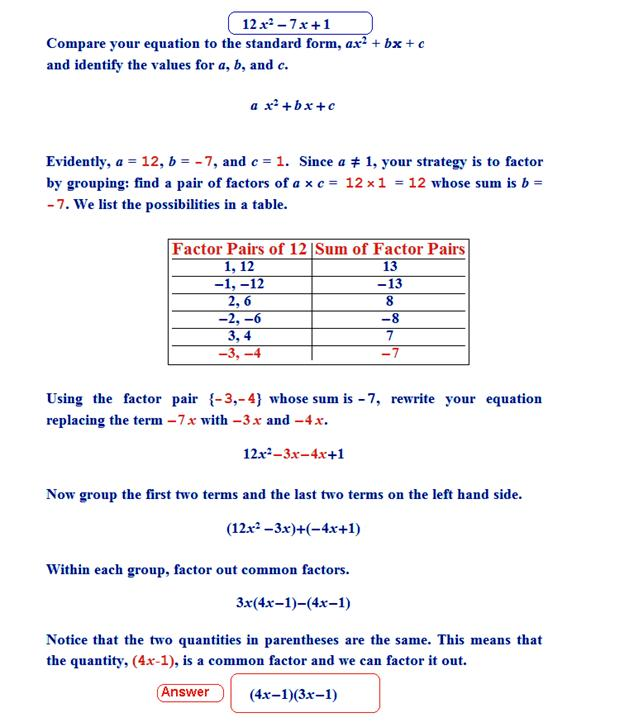(ii) 2x2 + 7x + 3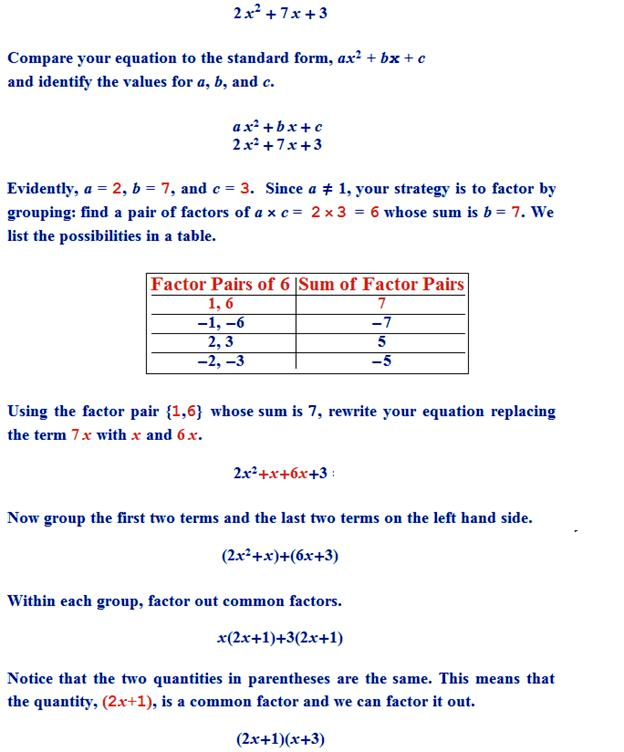(iii) 6x2 + 5x – 6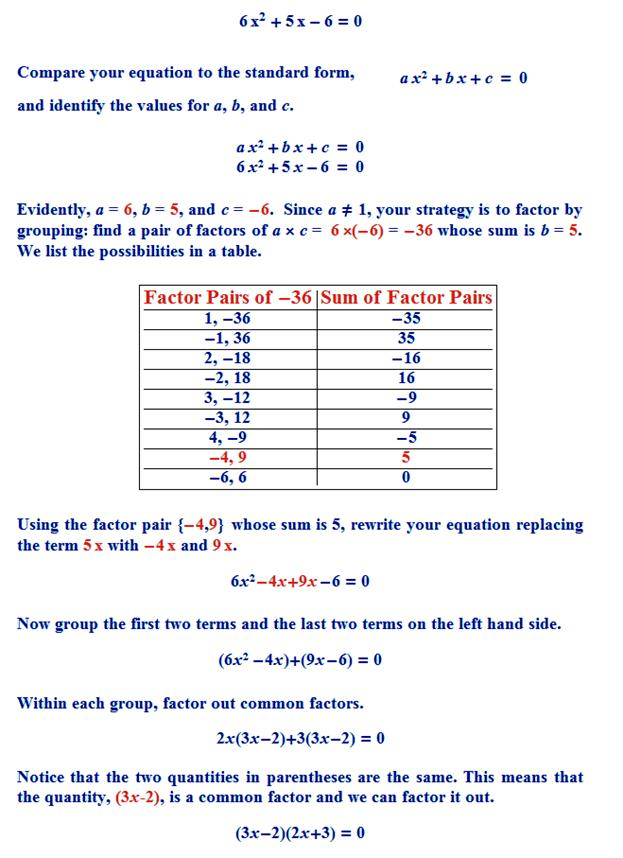(iv) 3x2 – x – 4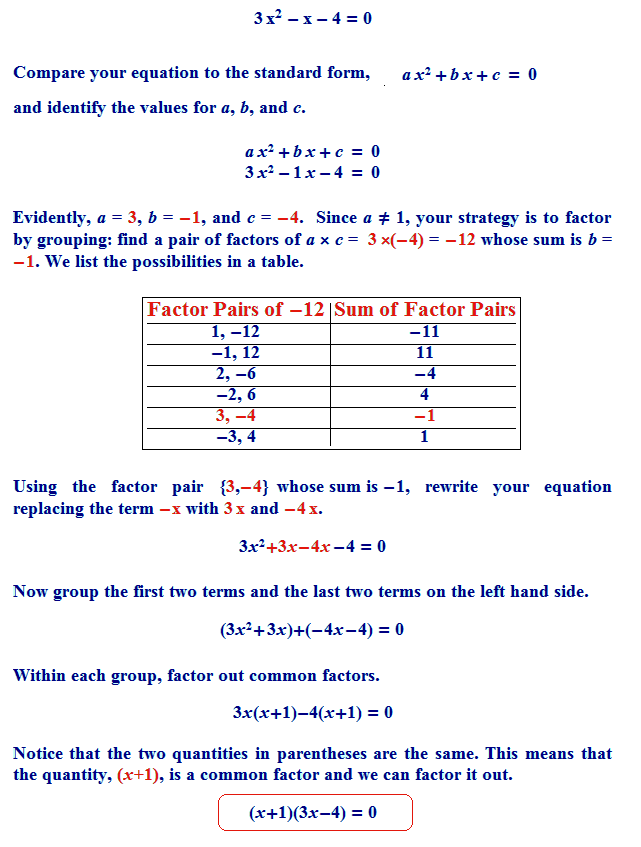Questoin 5. Factorise :
(i) x3 - 2x2 - x + 2
(ii) x3 - 3x2 - 9x - 5
(iii) x3 + 13x2 + 32x + 20
(iv) 2y3 + y2 - 2y - 1

Solution:(i) x3 - 2x2 - x + 2
Solution (i) Let take f(x) = x3 - 2x2 - x + 2
The constant term in f(x) is are ±1 and ±2
Putting x = 1 in f(x), we have
f(1) = (1)3 - 2(1)2 -1 + 2
= 1 - 2 - 1 + 2 = 0
According to remainder theorem f(1) = 0 so that (x - 1) is a factor of x3 - 2x2 - x + 2
Putting x = - 1 in f(x), we have
f(-1) = (-1)3 - 2(-1)2 –(-1) + 2
= -1 - 2 + 1 + 2 = 0
According to remainder theorem f(-1) = 0 so that (x + 1) is a factor of x3 - 2x2 - x + 2
Putting x = 2 in f(x), we have
f(2) = (2)3 - 2(2)2 –(2) + 2
= 8 -82 - 2 + 2 = 0
According to remainder theorem f(2) = 0 so that (x – 2 ) is a factor of x3 - 2x2 - x + 2

Here maximum power of x is 3 so that its can have maximum 3 factors
(ii) x3 - 3x2 - 9x - 5
Possible zeros are factors of ± constant term / coefficient of leading term
Here constant term is -5 and coefficient of leading term is 1
So that possible zeros will ±1 and ±5
Take f(x) = x3 - 3x2 - 9x - 5
Plug x = 1 we get
f(1) = (1)3 – 3(1)2 – 9(1) – 5
f(1) = 1 – 3 - 9 – 5
F(1) = -16 ≠ 0
So that (x-1) is not a factor of x3 - 3x2 - 9x - 5
Plug x = -1
f(-1) = (-1)3 – 3(-1)2 – 9(-1) – 5
f(1) = -1 – 3 + 9 – 5
F(1) = 0 so it a zero
x-1 = 0
x+1 = 0
So that (x+1) is a factor of x3 - 3x2 - 9x - 5
Divide the expression by x+1 we get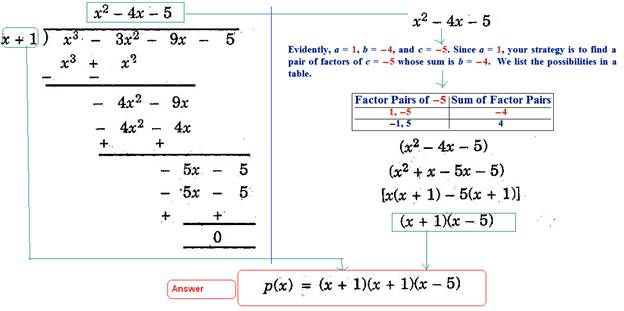(iii) x3 + 13x2 + 32x + 20
Here coefficient of leading term is 1 and constant term is 20
So possible zeros are factors of ± 20/1
So possible zeros are ±1 ,±2,±4,±5,±10, and ±20
And here all terms are positive so that zeros cannot positive
Plug x = -1
=>x3 + 13x2 + 32x + 20
=> (-1)3 + 13(-1)2 + 32(-1) + 20
=> -1+ 13 - 32 + 20
=> 0
So that (x+1) is a factor x3 + 13x2 + 32x + 20

Plug x = - 2

=>x3 + 13x2 + 32x + 20
=> (-2)3 + 13(-2)2 + 32(-2) + 20
=> -8+ 52 - 64 + 20
=> 0
So that (x+2) is a factor x3 + 13x2 + 32x + 20

As we have already find two zeros third zeros can 20 / 1*2 = 10
Plug x = 10 we get
Plug x = - 2

=>x3 + 13x2 + 32x + 20
=> (-10)3 + 13(-10)2 + 32(-10) + 20
=> -1000+ 1300 - 320 + 20
=> 0
So that (x+10) is a factor x3 + 13x2 + 32x + 20

As leading term has 3 powers so that there are only 3 roots are possible

(iv) 2y3 + y2 - 2y – 1
Here constant term is -1
Coefficient of leading term is 2
So possible zeros are ±1 ,±1/2
Plug y = 1
=>2y3 + y2 - 2y – 1
=>2(1)3 + (1)2 – 2(1) – 1
=> 2+ 1 -2-1
=>0
Here y= -1
Y+1 =0 so that
(y+1) is factor of 2y3 + y2 - 2y – 1

Plug y = -1
=>2y3 + y2 - 2y – 1
=>2(-1)3 + (-1)2 – 2(-1) – 1
=> - 2+ 1 + 2-1
=>0
Here y= 1
y - 1 =0 so that
(y - 1) is factor of 2y3 + y2 - 2y – 1

Plug y = ½
=>2y3 + y2 - 2y – 1
=>2(½)3 + (½)2 – 2(½) – 1
=> 2/8+ 1/4 -1-1
=>-3/2 ≠ 0
(y – ½) is factor of 2y3 + y2 - 2y – 1

Plug y = -½
=>2y3 + y2 - 2y – 1
=>2(-½)3 + (-½)2 – 2(-½) – 1
=> -2/8+ ¼ + 1-1
=>0
(y + ½) is factor of 2y3 + y2 - 2y – 1
Here y has max powers 3 so there are 3 possible factors
And our answer is (y-1)(y+1)(y+ -½)

### NCERT Books Free Pdf Download for Class 5, 6, 7, 8, 9, 10 , 11, 12 Hindi and English Medium

 Mathematics Biology Psychology Chemistry English Economics Sociology Hindi Business Studies Geography Science Political Science Statistics Physics Accountancy